# Texas Go Math Grade 2 Lesson 16.4 Answer Key Estimate Lengths to Solve Problems

Refer to our Texas Go Math Grade 2 Answer Key Pdf to score good marks in the exams. Test yourself by practicing the problems from Texas Go Math Grade 2 Lesson 16.4 Answer Key Estimate Lengths to Solve Problems.

## Texas Go Math Grade 2 Lesson 16.4 Answer Key Estimate Lengths to Solve Problems

Explore

Draw or write to solve the problem.
Ellen has _________ inches of ribbon now.

Carmen’s ribbon is ________ inches long now.
Ellen has 21 inches of ribbon now.
Carmen’s ribbon is 15 inches long now.

FOR THE TEACHER • Have children solve the following problem using the top workspace. Ellen has a blue ribbon that is 13 inches long. She gets a green ribbon that is 8 inches long. How many inches of ribbon does Ellen have now? Then ask children to use the bottom workspace to solve this problem: Carmen has a red ribbon that is 24 inches long. She cuts 9 inches off the ribbon. How many inches long is Carmen’s ribbon now?
Ellen has a blue ribbon that is 13 inches long. She gets a green ribbon that is 8 inches long
13+8=21
Therefore, Ellen has 21 inches of ribbon now.
Carmen has a red ribbon that is 24 inches long. She cuts 9 inches off the ribbon
Subtract 9 from 24
24-9=15
Therefore, Carmen has 15 inches of ribbon.

Math Talk
Mathematical Processes

How did you choose to solve the second problem? Explain.
I used the addition and subtraction methods to solve the second problem.

Model and Draw

Maria has these two flowers. If her orange flower is 6 inches tall, about how tall is her yellow flower? Which is the best estimate?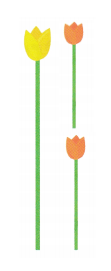Explanation:
Maria has these two flowers. Her orange flower is 6 inches tall, her yellow flower is about 2 times as tall as her orange flower.
So, 2 x 6 = 12
Therefore, the best estimate is 12 inches.

Share and Show

Circle the best estimate.

Question 1.
Eli builds a 2-foot long red cube train. He builds two blue cube trains. Use the picture of his cube trains to estimate the total length of the 3 trains.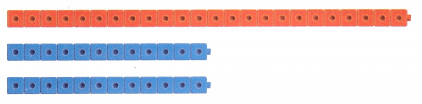Explanation:
Eli builds a 2-foot long red cube train. He builds two blue cube trains
The blue cube trains are about half of red train cube. So, blue cube train will be about 1 feet long
Add the lengths of red and two blue cube that is 2+1+1=4
Therefore, the estimate of total length of the 3 trains is about 4 feet long.

Question 2.
Dale has paper clips that are each about I inch long. About how long is the pencil?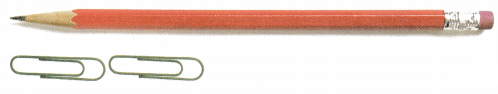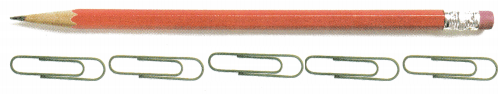Explanation:
Dale has paper clips that are each about I inch long
The pencil is about 5 paper clips long.
As 1 paper clip is 1 inch long So, the pencil is about 5 inches long.

Problem Solving

Circle the best choice.

Question 3.
Margie needs 5 pieces of string that are each about a foot long. Which length of string will be closest to the amount she needs?
25 inches of string
100 inches of string
70 inches of string
70 inches of string

Explanation:
Margie needs 5 pieces of string that are each about a foot long
As we know that 1 is feet is 12 inches long then 5 feet will be 5 x 12 = 60 inches
Therefore, the length of the string is 60 inches which is closest to 70 inches of string.

Question 4.
Louie makes a paper chain that is the about the same distance as the ceiling to the floor. About how long is Louie’s paper chain?

Question 5.
H.O.T. Multi-Step Kim has a toy train. Each train car is about 6 inches long. If Kim puts 8 train cars together, about how long will the train be in feet? Explain.4 feet

Explanation:
Kim has a toy train. Each train car is about 6 inches long.
If Kim puts 8 train cars together then the train will be 8 x 6 = 48 inches
As we know 1 feet is 12 inches then 48 inches is 4 feet
Therefore, the train is 4 feet long.

Question 6.
H.O.T. Jasper thinks the length of his book is about 1 inch. Clark thinks the length of the book is about 1 foot. Who made the better estimate? Explain.
Clark made the best estimate as we know that a book cannot be 1 inch long.

Question 7.
Reasoning Grayson has a white flower that is 1 foot long. She has a pink flower that is about half as long as the white flower. Which is the best estimate for the length of the pink flower?

Explanation:
Grayson has a white flower that is 1 foot long. She has a pink flower that is about half as long as the white flower
As we know that 1 feet is 12 inches
So, the pink flower will be half of 12 that is 6 inches long
Therefore, the best estimate is 5 inches as 6 inches is close to 5 inches.

Question 8.
Multi-Step Each section of a sidewalk is 2 feet long. Billy’s rope is about as long as three sections of sidewalk. Which is the best estimate for the length of Billy’s rope?

Explanation:
Each section of a sidewalk is 2 feet long
As we know 1 feet is 12 inches then 2 feet is equal to 24 inches
Billy’s rope is about as long as three sections of sidewalk
3 x 24 = 72  so, the rope is 72 inches long
Therefore, about 70 inches is the best estimate as 72 inches is close to 70 inches.

Question 9.
Texas Test Prep Tony has two pencils. If the yellow pencil is 8 inches long, about how long is the green pencil?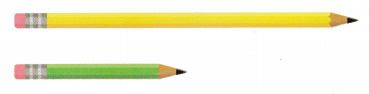Explanation:
Tony has two pencils. If the yellow pencil is 8 inches long
We can see that the green pencil is half of the length of yellow pencil
so, half of 8 is 4
Therefore, the green pencil is about 4 inches long.

TAKE HOME ACTIVITY • Ask your child to explain how he or she solved one of the problems in this lesson.

### Texas Go Math Grade 2 Lesson 16.4 Homework and Practice Answer Key

Circle the best choice.

Question 1.
Gary builds a 1-foot long red cube train. He builds two blue cube trains. Which estimate shows the total length of the trains?Explanation:
Gary builds a 1-foot long red cube train. He builds two blue cube trains
As we can see that blue cube train is half as long as red cube train then the blue cube train is half feet long
The red cube train is 1 feet long and the blue cube train is half feet long
So, the sum of 1 feet, half feet and half feet is 2 feet
Therefore, the total length of trains is 2 feet.

Problem Solving

Question 2.
Gail needs 3 pieces of string that are each about a foot long. Which length of string will be closest to the amount she needs?
15 inches of string
75 inches of string
5 inches of string
15 inches of string

Explanation:
Gail needs 3 pieces of string that are each about a foot long
As we know 1 feet is equal to 12 inches
12 is close to 15  the length of the string is closest to 15 inches
Therefore, the length of the string is closest to 15 inches.

Question 3.
Multi-Step Erin makes a paper chain. Each loop of the paper chain is about 3 inches long. Erin puts 8 loops of paper together. About how long will the paper chain be in feet? Explain.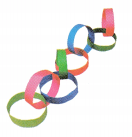2 feet

Explanation:
Erin makes a paper chain. Each loop of the paper chain is about 3 inches long
Erin puts 8 loops of paper together
so, 8 x 3 = 24 inches
As we know 12 inches makes 1 feet so, 24 inches will be 2 feet
Therefore, the paper chain is 2 feet long.

Lesson Check

Question 4.
Ethan has two pencils. The blue pencil is 6 inches long. About how long is the pink pencil?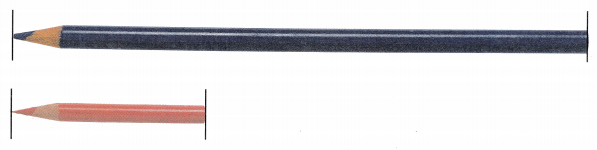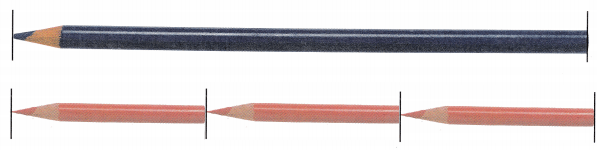Explanation:
Ethan has two pencils. The blue pencil is 6 inches long
As we can see that 3 pink pencils makes 1 blue pencil then the length of blue pencil is 3 times as pink pencil
6 divided by 3 is 2
Therefore, the pink pencil is 2 inches long.

Question 5.
Melinda has a sunflower that is 2 feet tall. She has a lily plant that is about half as tall. Which is the best estimate for the length of the lily plant?

Explanation:
Melinda has a sunflower that is 2 feet tall. She has a lily plant that is about half as tall
So, the length of the lily plant is 1 feet.

Question 6.
Multi-Step Daniela has a green polka dot ribbon that is 30 inches long. She uses 6 inches of the ribbon. Which is the best estimate of the length of the ribbon she has left?

Explanation:
Daniela has a green polka dot ribbon that is 30 inches long. She uses 6 inches of the ribbon
30-6=24 inches
The ribbon is 24 inches long
As we know 12 inches makes 1 feet then 24 inches will be 2 feet
Therefore, the length of the ribbon left with Daniela is 2 feet.

Question 7.
Stan has a piece of rope that is 13 inches long. He finds another piece of rope that is 18 inches long. About how many feet of rope does Stan have now?